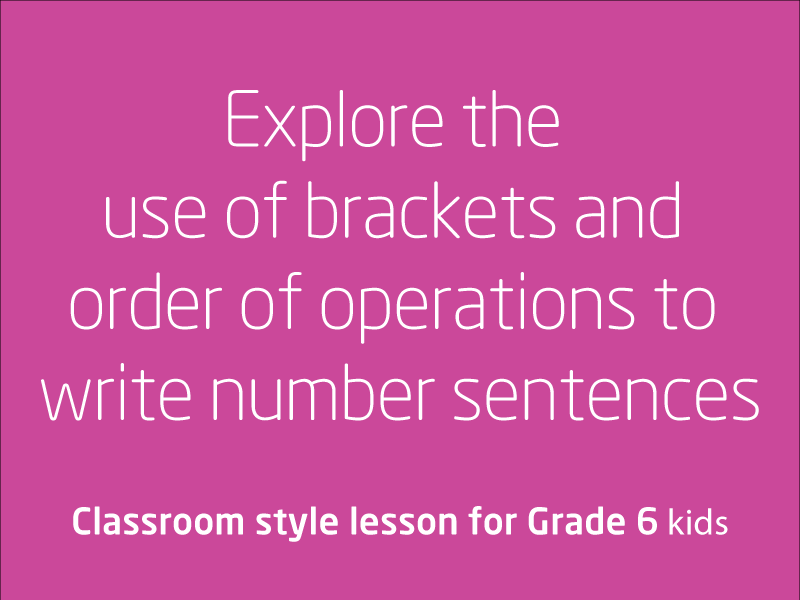# Free video lessons for Foundation to Grade 8 students### Fractions and decimals

In this video lesson, we will first be exploring some vocabulary related to Fractions and decimals. Then we will be demonstrating equivalence between fractions using drawings ...

1 videos
Intermediate difficulty
0 0 0### Addition and subtraction of fractions with the same or related denominators

In this video lesson, we will explore addition and subtraction of fractions with the same or related denominators.Elaborationsunderstanding the processes for adding and s...

1 videos
Intermediate difficulty
0 0 0### Finding a simple fraction of a quantity where the result is a whole number

In this video lesson, you will learn find a simple fraction of a quantity where a result is a whole number.Elaborationsrecognising that finding one-third of a quanti...

1 videos
Intermediate difficulty
0 0 0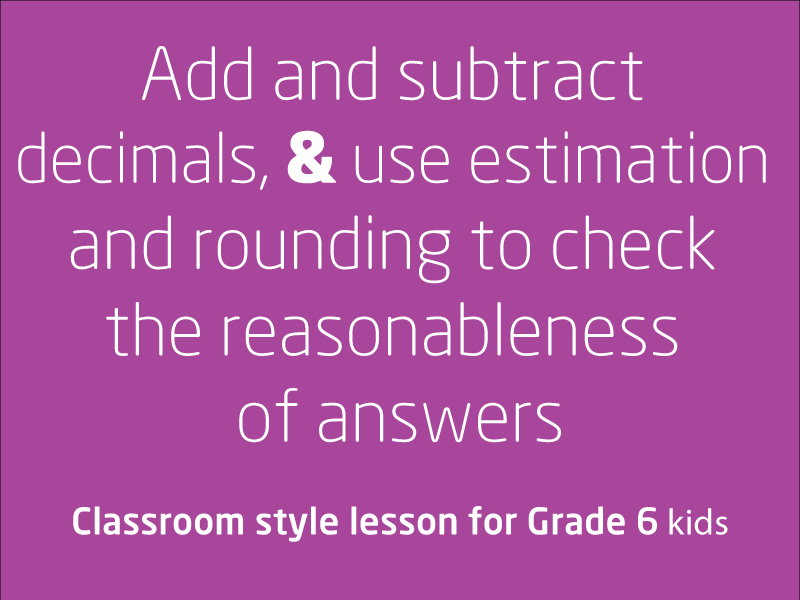### Add and subtract decimals, use estimation and rounding to check reasonableness

In this video lesson, we will be looking at adding and subtracting decimals and use estimation and rounding to check the reasonableness of answers.Elaborationsextending whole-numbe...

1 videos
Intermediate difficulty
0 0 0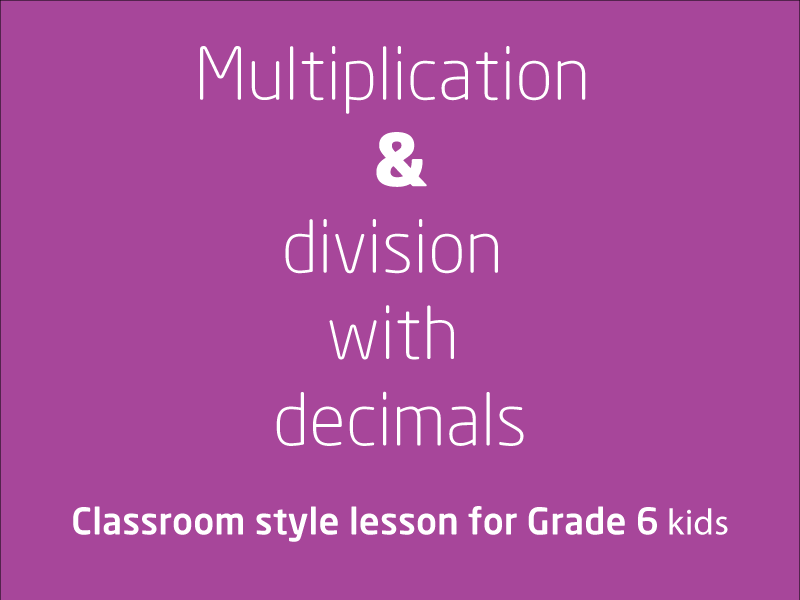### Multiplication and division with decimals

In this video lesson, we will explore multiplying decimals by whole numbers and perform divisions by non-zero whole numbers where the results are terminating decimalsElaborationsin...

1 videos
Intermediate difficulty
0 0 0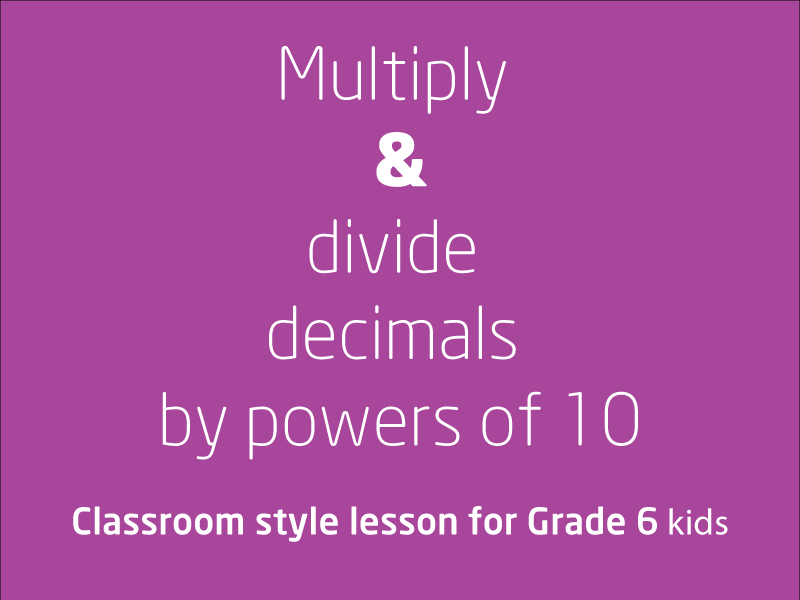### Multiply and divide decimals by powers of 10

In this lesson, we will learn how to multiply and divide decimals by powers of 10, this lesson will show you some quick trick to master this skill.

1 videos
Intermediate difficulty
0 0 0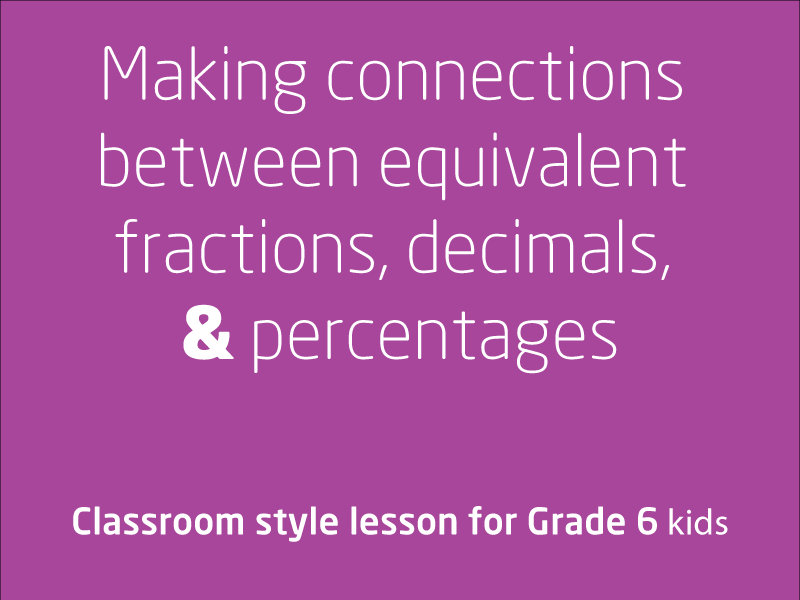### Equivalent fractions, decimals and percentages

In this video lesson, we will explore making connections between equivalent fractions, decimals, and percentagesElaborationsElaborationsconnecting fractions, decimals, and per...

1 videos
Intermediate difficulty
0 0 0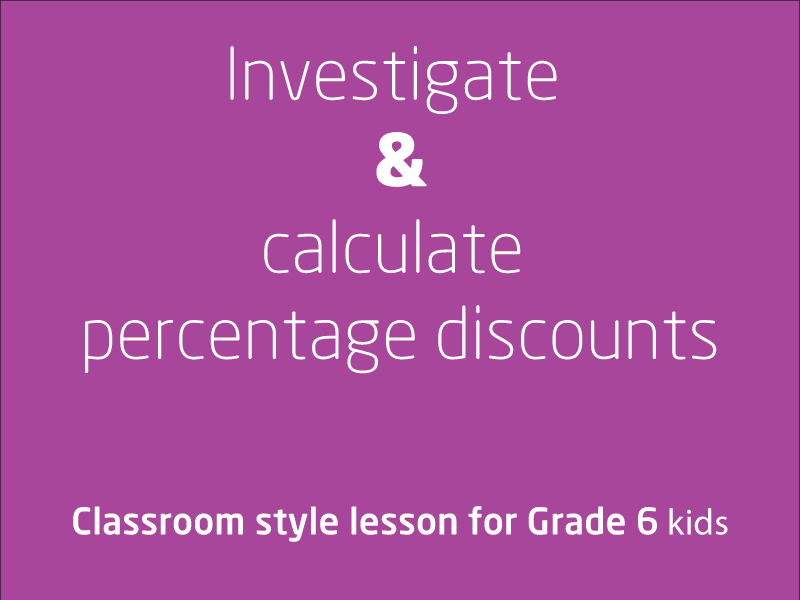### Investigate and calculate percentage discounts

In this video lesson, we will be using some authentic data to calculate prices on sale goods, and thus investigating and calculating percentage discounts.

1 videos
Intermediate difficulty
0 0 0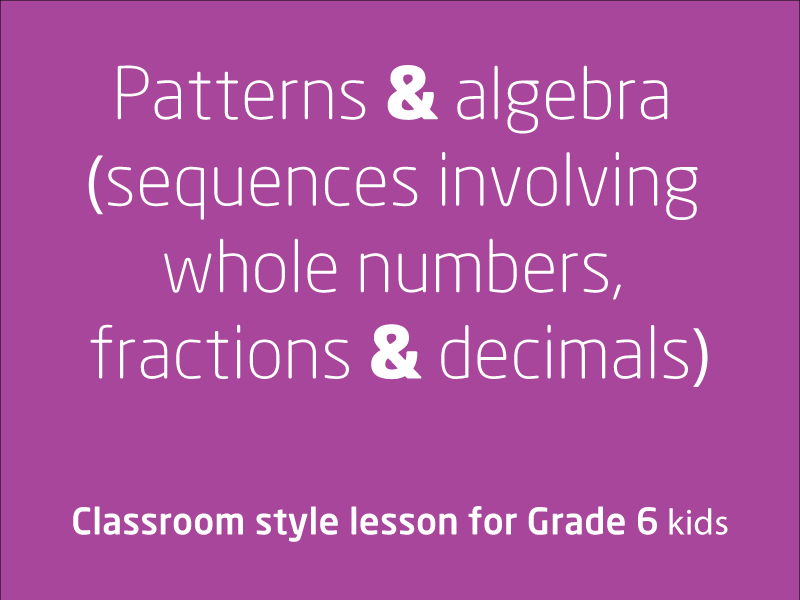### Patterns and algebra (sequences involving whole numbers, fractions and decimals)

In this video lesson we will continue and create sequences involving whole numbers, fractions and decimals, and describe the rule used to create the sequence.

1 videos
Intermediate difficulty